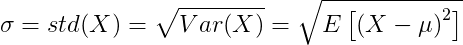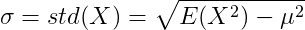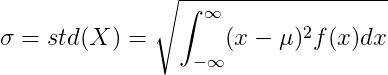# Standard Deviation

In statistics, the standard deviation (SD, also represented by the Greek letter sigma σ or the Latin letter s) is a measure that is used to quantify the amount of variation or dispersion of a set of data values. A low standard deviation indicates that the data points tend to be close to the mean (also called the expected value) of the set, while a high standard deviation indicates that the data points are spread out over a wider range of values.

Contents

### Standard deviation definition formula

The standard deviation is the square root of the variance of random variable X, with mean value of μ.From the definition of the standard deviation we can get:### Standard deviation of continuous random variable

For continuous random variable with mean value μ and probability density function f(x):or:### Standard deviation of discrete random variable

For discrete random variable X with mean value μ and probability mass function P(x):or: